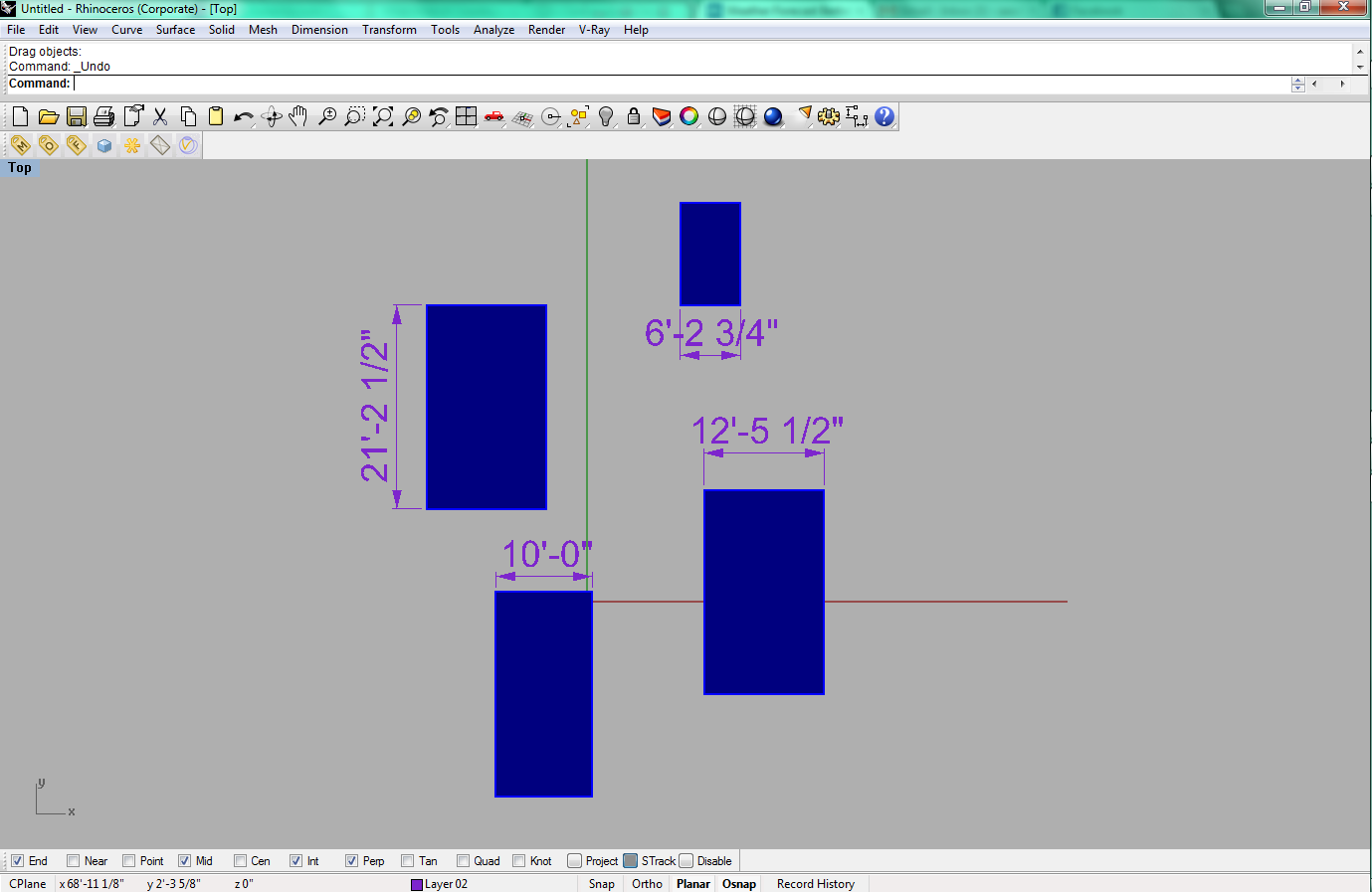Units
Units are the measurement system of the model which are used to create precise geometry.
Part of Rhinoceros , Rhinoceros for OSX
Part Type Interface
Screenshot## Units in Rhino

The default units in Rhino are determined by the size of the model (which is chosen when Rhino is first opened). Units are useful when entering exact measurements for a geometric form such as a line or circle radius, or the height of a box.

There are many different ways to enter units. Once a unit has been set, a measurement can be entered as a whole number (positive or negative), decimal number, scientific notation, or fraction. For example, if the units are set to inches, five and a half inches can simply be entered as "5.5". For angle units, the default unit is degrees unless otherwise specified. When prompted for a point, the coordinates refer to (x, y, z). For more ways of entering and using different types of units, see the Resources.

## Changing Units

To change the model's units, use the "Units" command to pull up the Document Properties window.

Model Units
Choose the unit system used in the model. If the unit system is changed, Rhino may ask if the model should be automatically scaled to match the change.
Tolerances
Tolerance in units used when creating geometry that cannot be absolutely accurate. Smaller values for tolerances creates more accurate geometry.
Distance Display
The options for how measurements are displayed in the status bar for distance and length commands.
Display precision
Sets the number of decimal places for the distance display.

## Resources

Unit systems
Different types of unit systems and how to enter them into Rhino